# Copy the following on a squared paper. A square paper is what you would have used in your arithmetic notebook in earlier classes. Then complete them such that the dotted line is the line of symmetry.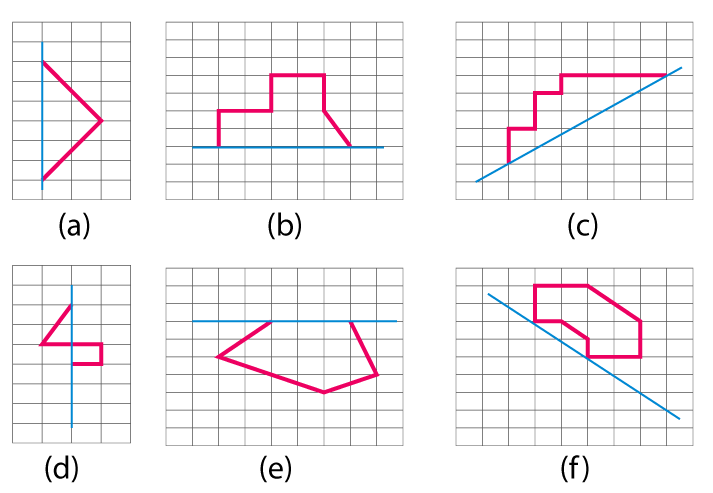Solution:

To make the dotted line a line of symmetry the given figure may be drawn as shown below

(a)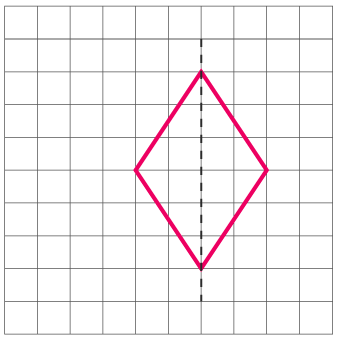(b)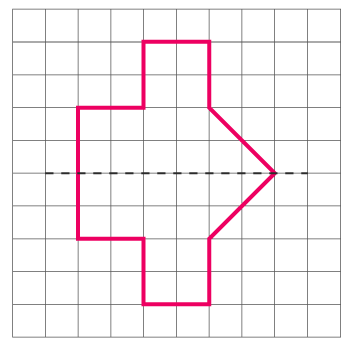(c)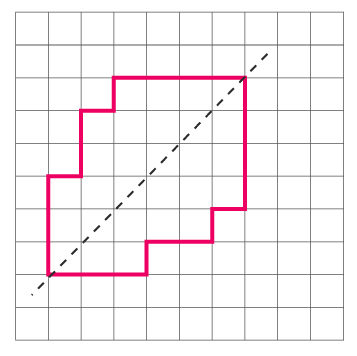(d)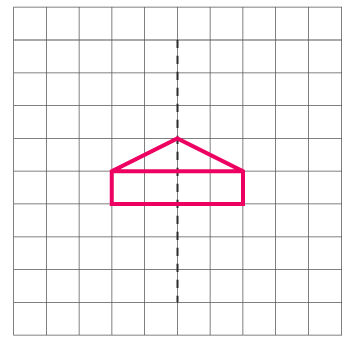(e)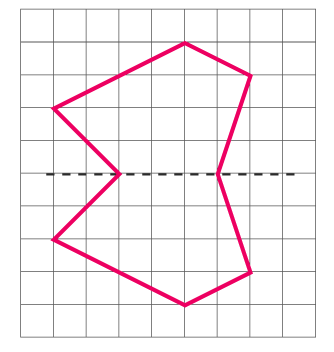(f)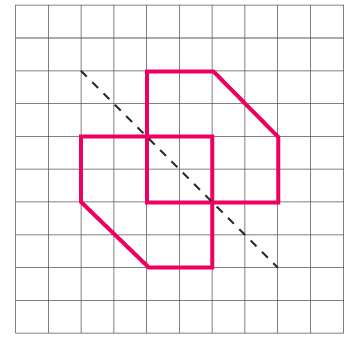(0)(0)